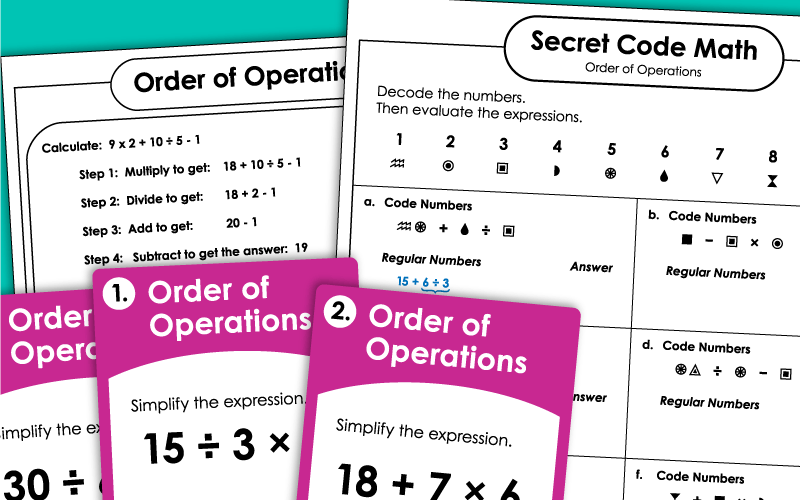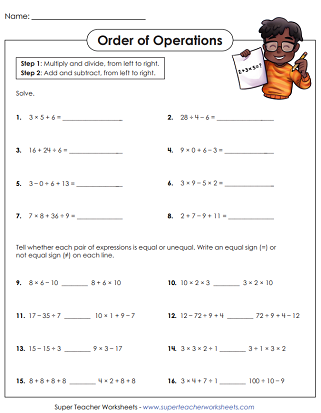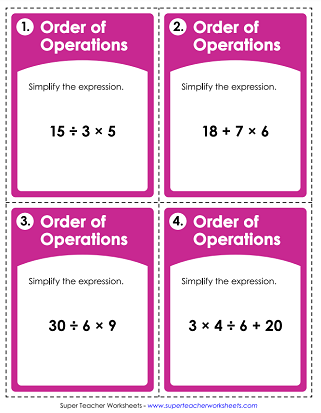# Order of Operations (Basic: No Parentheses or Exponents)

This page contains very basic Order of Operations worksheets. The expressions and equations on these worksheets do not include parentheses or exponents.This worksheet requires students to add, subtract, multiply, and divide. There are no exponents or parentheses in these problems.
example: 7 × 8 + 36 ÷ 9
Use knowledge of Order of Operations to solve the equations. THen match the answers with the letters at the bottom of the page to find the answer to a silly riddle. What's a cat's favorite color? Purrr-ple.
example: 24 ÷ 2 + 20 - 20 ÷ 4
These task cards have expressions. Students use the order of operations to simplify. Recommended for classroom scavenger hunts, peer practice sessions, document camera work, or exit slips.
example: 3 × 4 ÷ 6 + 20
This basic practice page includes a box which shows students a sample order of operations problem step-by-step.
Use the symbol key to decode the expressions. Then find the answers.
example: 8 × 9 + 4
Color the circles with the problems, as well as the answers. If you correctly complete each one, you'll be able to navigate the maze from start to finish.
More Order of Operations

## Sample Worksheet ImagesMy Account
Site Information